Neural Network - Perceptron                                 Home : www.sharetechnote.com

Perceptron - Experiment - |x|

In this page, I would do some very basic experiment with a dataset that I created (not a collected data) and try to show you overall procedure on how you design a simple neural network for a given data.

Step 1 : Take a overall distribution of the data plotted as below and try to come up with some idea what kind of neural network you can use. I use the following code to create this dataset. It would not be that difficult to understand the characteristics of dataset from the code itself, but don't spend too much time and effort to understand this part. Just use it as it is.

rng(1);

full_data = [];

xrange = 5;

for i = 1:150

x = xrange * rand(1,1);

y = xrange * rand(1,1);

if y >= 2*abs(x-xrange/2)

z = 1;

else

z = 0;

end

full_data = [full_data ; [x y z]];

end

In this case, only two parameters are required to specify a specific data. That's why you can plot the dataset in 2D graph. Of course, in real life situation this would not be a frequent case where you can have this kind of simple dataset, but I would recommend you to try with this type of simple dataset with multiple variations and understand exactly what type of variation you have to make in terms of neural network structure to cope with those variations in data set.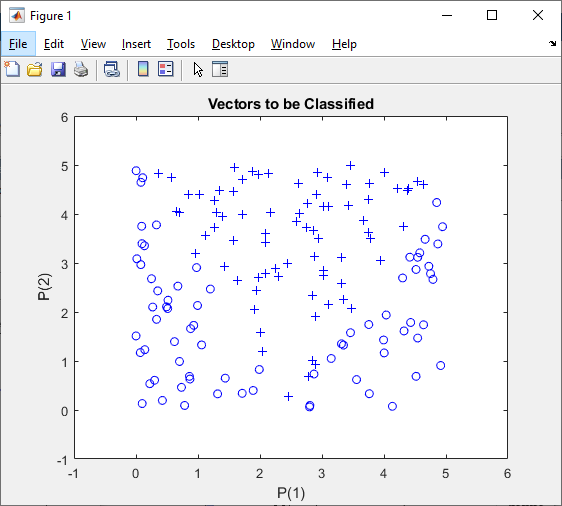Just taking a quick look at the data, I see there is only two categories of data labeled as '+' and 'o'. So I would need only two input in my neural network.

Then next question is 'would it be possible to classify these two groups of data by a single linear line ?

Obviously Not. It mean that single perceptron and single layer would not solve this classification issue.

Then next question is 'how many output you would need for this classification ? I think I can do this with only one output since there are only two categories.

NOTE : You may use more than one outputs for this classification depending on how you map the output value(s) to each categories of this dataset, but if I can do with one output, what is point of using multiple outputs.

Step 2 : Determine the structure of Neural Nework.

At step 1, I concluded that I would need two inputs and one outputs in the neural network, but the number of layers should be greater than 1. The simplest neural network structure that meets this criteria would be as follows. This network has two inputs and one hidden layer made up of two cells and one output layer made up of one cell. It looks as shown below.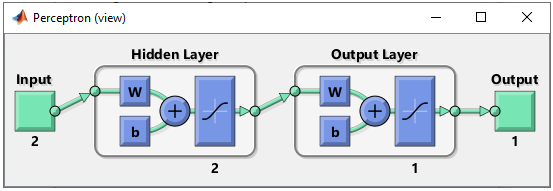This structure can be created by following code. For further details of meaning of these code, refer to this tutorial.

net = perceptron;

net.numLayers = 2;

net.layers{1}.name = 'Hidden Layer';

net.layers{1}.transferFcn = 'tansig'; % try other transfer function referring here and see how it works.

net.layers{1}.dimensions = 2;

net.layers{2}.name = 'Output Layer';

net.layers{2}.transferFcn = 'tansig'; % try other transfer function referring here and see how it works.

net.layers{2}.dimensions = 1;

Then define the connection between each layers. If you want further details on meaning of these code, refer to this tutorial.

net.layerConnect = [0 0;...

1 0];

net.outputConnect = [0 1];

net.biasConnect = [1;...

1];

net = configure(net,[0; 0],);

Now Initialize the weight and bias of every perceptrons (neurons). If you want further details on meaning of these code, refer to this tutorial.

net.b{1} =  [1; ...

1];

net.b{2} = 1;

wi = [1 -0.8; ...

1 -0.8];

net.IW{1,1} = wi;

wo = [1 -0.8];

net.LW{2,1} = wo;

Step 3 : Train the network.

Training a network is done by a single line of code as follows.

[net,tr] = train(net,pList,tList);

Just running this single line of code may nor may not solve your problem. In that case, you may need to tweak the parameters involved in the training process. First tweak the following parameters and try with different activation functions in step 2.

net.performFcn = 'mse';

net.trainFcn = 'trainlm';

net.trainParam.epochs =1000;

net.trainParam.goal = 10^-10;

The result in this example is as shown below.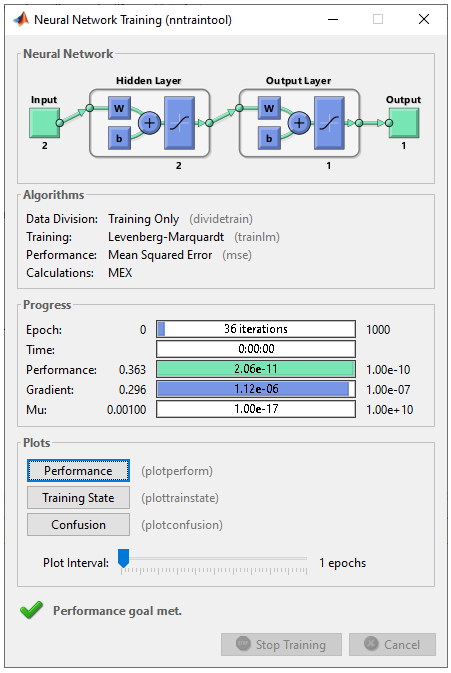As shown on the left, it seems that the network did relatively good job separating the data set into two groups with two lines. As shown on the right, out of 100 test dataset only 3 got the wrong prediction.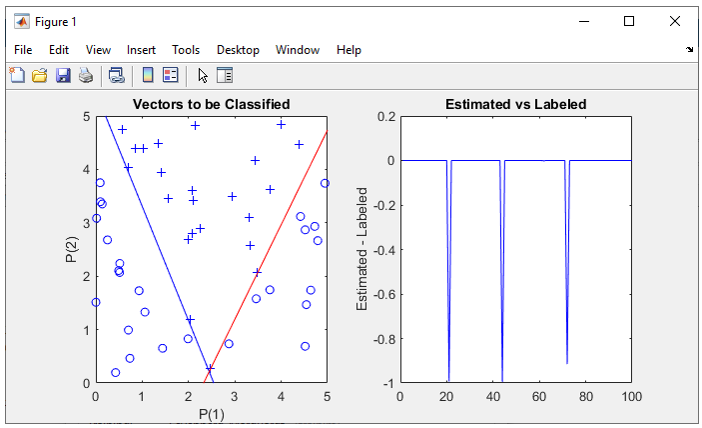All of the following plots are showing how the training process reaches the target at each iteration. I would not explain on these plots.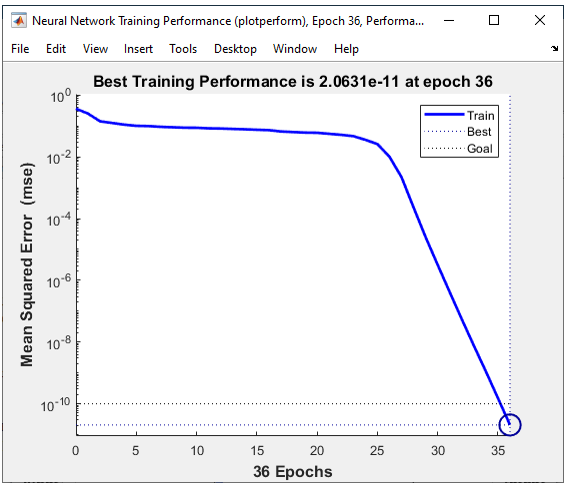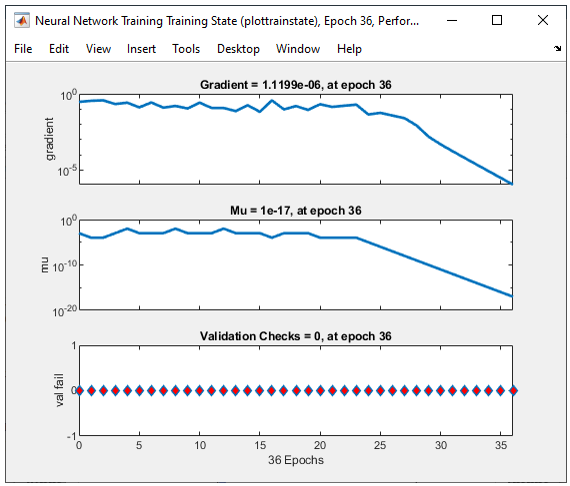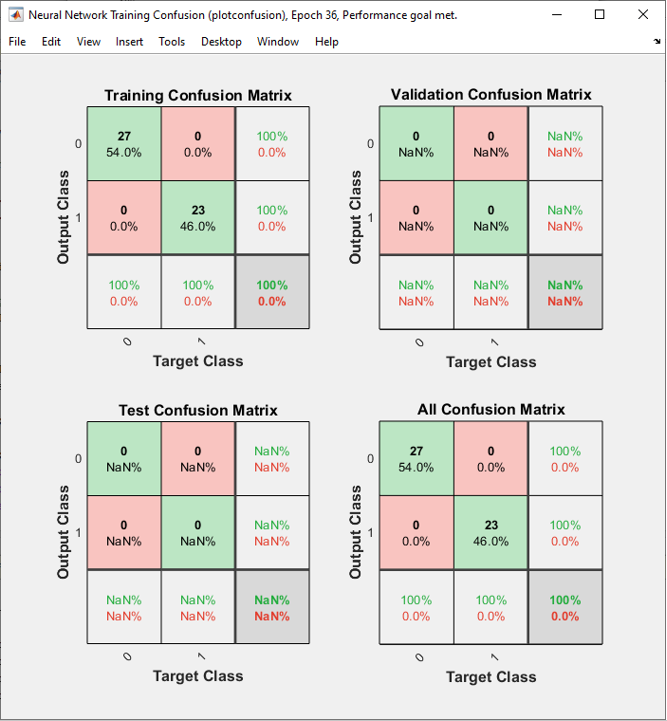% Generate Data Set rng(1); full_data = [];   xrange = 5; for i = 1:150         x = xrange * rand(1,1);     y = xrange * rand(1,1);          if y >= 2*abs(x-xrange/2)         z = 1;     else         z = 0;     end          full_data = [full_data ; [x y z]];      end         % Split the dataset into Training set and Test Set data_Train = full_data(1:50,:); data_Test = full_data(51:150,:);     % Initialize Input Vector with Training Dataset pList = [data_Train(:,1)'; data_Train(:,2)']; tList = data_Train(:,3)';   figure(1); plotpv([full_data(:,1)'; full_data(:,2)'],full_data(:,3)'); axis([-1 6 -1 6]);   figure(2);   % Build a Neural Net subplot(1,2,1);   plotpv(pList,tList); axis([-1 6 -1 6]);   net = perceptron;   net.numLayers = 2;   net.layers{1}.name = 'Hidden Layer'; net.layers{1}.dimensions = 2;   net.layers{2}.name = 'Output Layer'; net.layers{2}.dimensions = 1;   net.layers{1}.transferFcn = 'tansig'; net.layers{2}.transferFcn = 'tansig';   net.layerConnect = [0 0;...                            1 0]; net.outputConnect = [0 1];       net.biasConnect = [1;1];   net = configure(net,[0; 0],);   net.b{1} =  [1; ...              1]; net.b{2} = 1; wi = [1 -0.8; ...       1 -0.8]; net.IW{1,1} = wi;   wo = [1 -0.8]; net.LW{2,1} = wo;     % Perform Training   net.performFcn = 'mse'; net.trainFcn = 'trainlm'; net.trainParam.epochs =1000; net.trainParam.goal = 10^-10; view(net);   [net,tr] = train(net,pList,tList);     % Plot Training Result plotpc(net.IW{1,1},net.b{1}) axis([-1 6 -1 6]);   pList = [data_Test(:,1)'; data_Test(:,2)']; tList = data_Test(:,3)';     subplot(1,2,2);   y = net(pList); plot(y-tList,'b-'); ylabel('Estimated - Labeled'); title('Estimated vs Labeled');

Next Step :